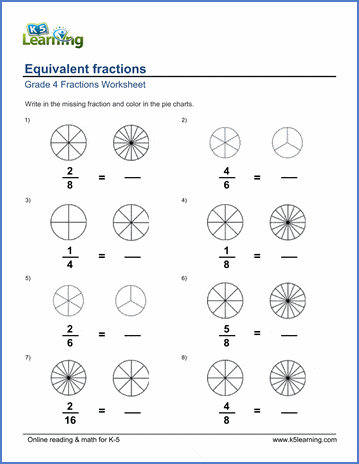# Writing equivalent fractions

## Worksheets: Writing equivalent fractions

Below are six versions of our grade 4 fractions worksheet on writing equivalent fractions. Students must fill in the missing numerator or denominator to make the two fractions shown equivalent.  All fractions are proper fractions. These worksheets are pdf files.## More fractions worksheets

Explore all of our fractions worksheets, from dividing shapes into "equal parts" to multiplying and dividing improper fractions and mixed numbers.

## What is K5?

K5 Learning offers reading and math worksheets, workbooks and an online reading and math program for kids in kindergarten to grade 5.  We help your children build good study habits and excel in school.